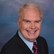# Math Solutions: Helpful Math Hints And CalculationsBy Daniel Theobald, "Wastewater Dan"

Mathematical calculations can be a challenge for even the most skilled water and wastewater operator. The formulas for chemical dosing, conversion, filtration, pounds, velocity, volume, and other everyday problems vary, of course, but there are a scant number of basic ground rules that can help make sure your math solution is legitimate regardless of the calculation you’re performing.

The helpful hints below are the summary of my 25-plus years experience and the prowess of other masters.

1. Learn what a formula entails, not merely when it is utilized.
2. Use drawings and unit labels throughout your calculation to help you designate the appropriate math operator, such as addition, subtraction, multiplication, or division.
3. Always convert percentages to decimals to position into a formula and convert decimals to percentages as required of the problem for an appropriate solution.
4. Make sure the units accord with the problem you are attempting to figure out.
5. Pertaining to Conversion calculations, for flow conversions: pay close attention to the units in which flow is expressed, in increments — millions as million-gallons (MG) or million gallons per day (MGD); or time as minutes (gallons per minute [gpm]), hours (gallons per hour [gph]), or days (gallons per day [gpd]), and so forth.
6. Pertaining to Volume calculations, for entering into the formula to compute cubic feet: convert “inches” to “feet”;  or convert “yards” to “feet”; or convert “fractions” to “decimal equivalent”;  otherwise, you will almost invariably end up with the incorrect solution.
7. Pertaining to Poundage calculations, for working out the pounds of an item such as biochemical oxygen demand (BOD) or total suspended solids (TSS) in a flowing stream or in a tank: determine the type of problem and exact requirement to solve the problem.
8. Constantly trust your skepticism. If the answer does not seem right, check that you selected the appropriate formula, units, and that the math problem is solved accurately.
9. Always work out each problem twice or until you arrive at the same answer twice.

In doing everything to master the competence of you and/or your colleagues, consider using this tool as another source for ultimate proficiency.

This tool for operators, represented through video tutorials, encompasses mathematical templates on Helpful Math Hints And Calculations of math word problems computation solutions.

The tool also provides definitions, explanations, and alternative applications for the calculations beyond the specific examples presented. In addition to operators, it may also serve educators, engineers, regulators, and others.

Representative Helpful Math Hints And Calculations of arithmetic word problems and exact step-by-step solutions are categorized as Conversions, Volume, and Poundage, which are available to download and view below.

Wastewater Conversions Word Problems and Solutions:

Conversion of pump stroke into gallons:

Conversion of gallons into cubic yards:

Convert flow from milliliters per minute (mL/min) to GPM:

Wastewater Volume Word Problems and Solutions:

Calculate aeration volume (MG):

Calculate cubic feet of circular tank:

Calculate liters in treatment tank:

Wastewater Poundage Word Problems and Solutions:

Calculate pounds (lbs) TSS effluent per day:

Calculate pounds (lbs) MLSS in activated sludge system:

Calculate pounds (lbs) of chlorine used in one year:

Playlist Calculations:

Playlist of all 35-plus Conversions calculations

Playlist of all 20-plus Volume calculations

Playlist of all 45-plus Poundage calculations

Playlist of all 165-plus Math Solutions

This is the Helpful Math Hints And Calculations presentation in my series of “Math Solutions.” If you have specific wastewater math queries, please submit a question.